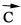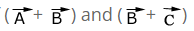# Commutative Law And Associative Law Of Vector Addition

Consider two vectors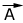and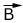Let these two vectors represent two adjacent sides of a parallelogram. We construct a parallelogram OACB as shown in the diagram. The diagonal OC represents the resultant vector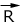From above figure it is clear that:
This fact is referred to as the commutative law of vector addition.

Consider three vectors: